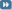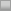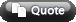View Single Post09-13-2009, 10:24 PM   #3
jason1990
old hand

Join Date: Sep 2004
Posts: 1,876
Re: How to include LaTeX images in your posts

Quote:
 Originally Posted by jason1990To post LaTeX formulas as images in your posts, use the following syntax: [img]http://latex.codecogs.com/gif.latex?insert LaTeX code here[/img]For example, the LaTeX image: is produced by the syntax: [img]http://latex.codecogs.com/gif.latex?\Phi(x) = \frac1{\sqrt{2\pi}} \int_{-\infty}^x e^{-u^2/2} \, du[/img]
As I am writing this, the above LaTeX image is not visible. In fact, none of the LaTeX images which use this syntax are visible, because codecogs.com is down. Entire threads are now unreadable.

Of course, this is the risk we run when we rely on an outside site for our posting needs, instead of having a 2+2 owned LaTeX editor at our disposal.

FWIW, I have found another external LaTeX editor, which therefore gives us an alternative syntax:
[img]http://mathstat.uohyd.ernet.in/equationeditor/rawequation.php?insert LaTeX code here[/img]
This one seems to be working (at least for now):